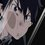# Try to solve it 2

$\large \lim_{x\to1} \frac{x+x^2+x^3+\ldots +x^n-n}{\sqrt x-1} = \ ?$Note by Abdulrahman El Shafei
5 years, 10 months ago

This discussion board is a place to discuss our Daily Challenges and the math and science related to those challenges. Explanations are more than just a solution — they should explain the steps and thinking strategies that you used to obtain the solution. Comments should further the discussion of math and science.

When posting on Brilliant:

• Use the emojis to react to an explanation, whether you're congratulating a job well done , or just really confused .
• Ask specific questions about the challenge or the steps in somebody's explanation. Well-posed questions can add a lot to the discussion, but posting "I don't understand!" doesn't help anyone.
• Try to contribute something new to the discussion, whether it is an extension, generalization or other idea related to the challenge.

MarkdownAppears as
*italics* or _italics_ italics
**bold** or __bold__ bold
- bulleted- list
• bulleted
• list
1. numbered2. list
1. numbered
2. list
Note: you must add a full line of space before and after lists for them to show up correctly
paragraph 1paragraph 2

paragraph 1

paragraph 2

[example link](https://brilliant.org)example link
> This is a quote
This is a quote
    # I indented these lines
# 4 spaces, and now they show
# up as a code block.

print "hello world"
# I indented these lines
# 4 spaces, and now they show
# up as a code block.

print "hello world"
MathAppears as
Remember to wrap math in $$ ... $$ or $ ... $ to ensure proper formatting.
2 \times 3 $2 \times 3$
2^{34} $2^{34}$
a_{i-1} $a_{i-1}$
\frac{2}{3} $\frac{2}{3}$
\sqrt{2} $\sqrt{2}$
\sum_{i=1}^3 $\sum_{i=1}^3$
\sin \theta $\sin \theta$
\boxed{123} $\boxed{123}$

Sort by:

Without L'Hôpital's rule

Rationalize the denominator by multiplying the expression with $\frac{\sqrt{x} + 1} {\sqrt{x} + 1}$. Distribute the $n$ and rewrite the expression as

$\lim _{ x \to 1 } \frac{ (x - 1) + (x^{2} - 1) + (x^{3} - 1) + \cdots + (x^{n} - 1) }{x -1} \cdot (\sqrt{x} + 1)$

$= \lim _{ x \to 1 } \left( \frac{ x - 1 } { x -1 } + \frac{ x^{2} - 1 } { x -1 } + \frac{ x^{3} - 1 } { x -1 } + \cdots + \frac{ x^{n} - 1 } { x -1 }\right) \cdot (\sqrt{x} + 1)$

$= \lim _{ x \to 1 } \left( (1) + (1 + x) + (1 + x + x^{2}) + (1 + x + x^{2} + x^{3}) + \cdots + (1 + x + x^{2} + x^{3} + \cdots + x^{n-1}) \right) \cdot (\sqrt{x} + 1)$

Now solve by substitution:

$= ( 1 + 2 + 3 + \cdots + n) \cdot (1 + 1) = \boxed{n(n+1)}$

- 5 years, 10 months ago

Amazing solution! :)

- 5 years, 6 months ago

$n^{2}+n$???

- 5 years, 10 months ago

Did you use L.Hospital's Rule ?

- 5 years, 10 months ago

Yes. Can u prove the L.hospital rule?

- 5 years, 10 months ago

n(n+1)

- 5 years, 10 months ago

Did you use L.Hospital's Rule ?

- 5 years, 10 months ago

Yes. However I cannot post my solution. My Latex skills s*cks. Sorry.

- 5 years, 10 months ago

n(n+1) May be thats the answer. If it is correct please notify.

- 5 years, 10 months ago

Did you use L.Hospital's Rule ?

- 5 years, 10 months ago

n(n+1)

- 5 years, 10 months ago

Did you use L.Hospital's Rule ?

- 5 years, 10 months ago

n(n+1)

- 5 years, 1 month ago

Since this is an indeterminate form of the $\dfrac{0}{0}$ form, we can simply use the L.Hospital's Rule to evaluate the given limit, which is as you follow:

$\begin{array}{} & \lim_{x\to1} \dfrac{x+x^2+x^3+\ldots +x^n-n}{\sqrt x-1} \\ & = \lim_{x \to 1} \dfrac{1+2x+3x^2+\ldots +nx^{n-1}}{\dfrac{1}{2 \sqrt x}} \\ & = n(n+1) \end{array}$

- 5 years, 10 months ago

There is an answer without using L.Hospital's Rule ,....can u find it ?

- 5 years, 10 months ago

There will be no difference in the answer whether you solve it using L.Hospital's rule or not. But yeah, there obviously exists a way to evaluate it without using L.Hospital's rule, as Pranshu did. And notice that the answer is still the same.

- 5 years, 10 months ago

We could just use L'Hopital's rule but sometimes we can search about another answer ...I was looking for an approach that does not use this rule and I am sorry I didn't mention , but your solution still elegant :))

- 5 years, 10 months ago

n(n+1)

- 3 years, 2 months ago